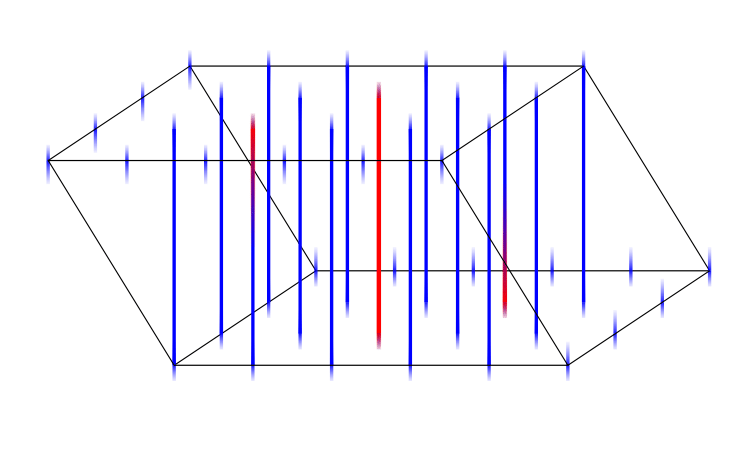# High-dimensional holeyominoes

### Abstract

What is the maximum number of holes enclosed by a $d$-dimensional polycube built of $n$ tiles? Represent this number by $f_d(n)$. Recent results show that $f_2(n)/n$ converges to 12. We prove that for all $d \geq 2$ we have $f_d(n)/n \to (d-1)/d$ as $n$ goes to infinity. We also construct polycubes in $d$-dimensional tori with the maximal possible number of holes per tile. In our proofs, we use metaphors from error-correcting codes and dynamical systems.

Publication
The Electronic Journal of CombinatoricsA fundamental domain for a linear flow used to define toric polycubes. A contiguous portion of the flow line is highlighted in red.